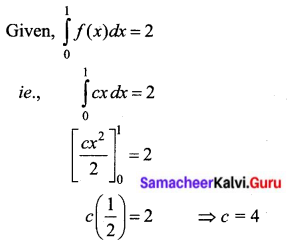Students can download 12th Business Maths Chapter 2 Integral Calculus I Ex 2.8 Questions and Answers, Samacheer Kalvi 12th Business Maths Book Solutions Guide Pdf helps you to revise the complete Tamilnadu State Board New Syllabus and score more marks in your examinations.

## Tamilnadu Samacheer Kalvi 12th Business Maths Solutions Chapter 2 Integral Calculus I Ex 2.8

I. Using second fundamental theorem, evaluate the following:

Question 1.
$$\int_{0}^{1} e^{2 x} d x$$
Solution: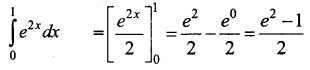Question 2.
$$\int_{0}^{\frac{1}{4}} \sqrt{1-4 x} d x$$
Solution: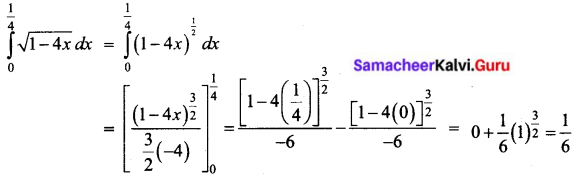Question 3.
$$\int_{1}^{2} \frac{x d x}{x^{2}+1}$$
Solution: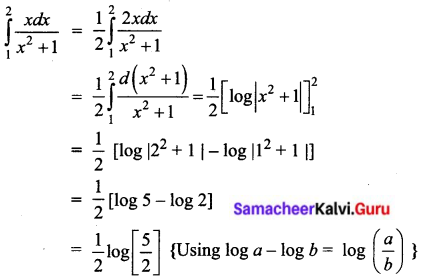Question 4.
$$\int_{0}^{3} \frac{e^{x} d x}{1+e^{x}}$$
Solution: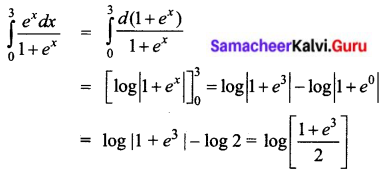Question 5.
$$\int_{0}^{1} x e^{x^{2}} d x$$
Solution: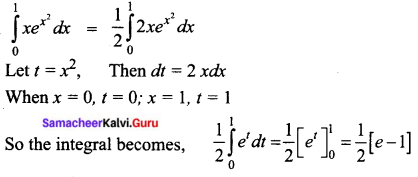Question 6.
$$\int_{1}^{e} \frac{d x}{x(1+\log x)^{3}}$$
Solution: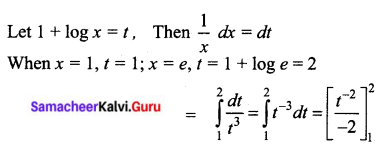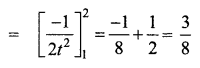Question 7.
$$\int_{-1}^{1} \frac{2 x+3}{x^{2}+3 x+7} d x$$
Solution: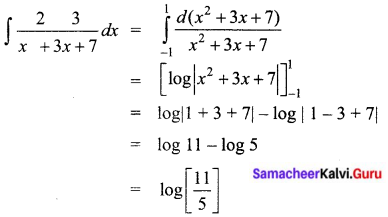Question 8.
$$\int_{0}^{\frac{\pi}{2}} \sqrt{1+\cos x} d x$$
Solution: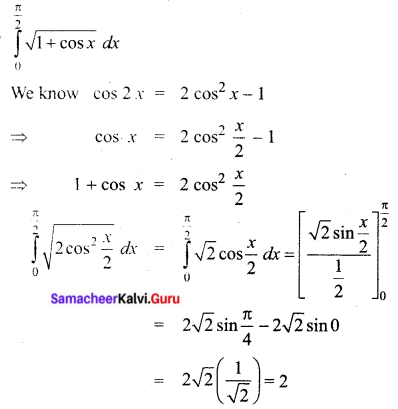Question 9.
$$\int_{1}^{2} \frac{x-1}{x^{2}} d x$$
Solution: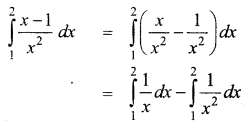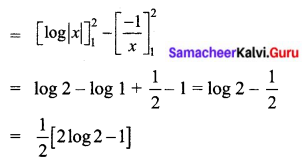II. Evaluate the following:

Question 1.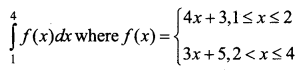Solution: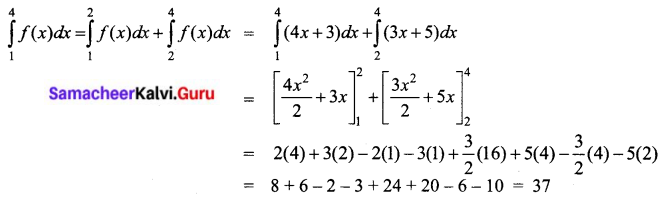Question 2.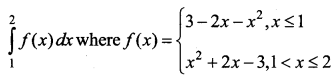Solution: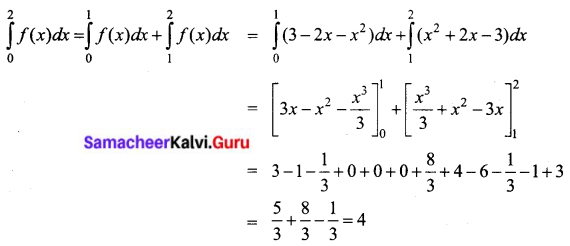Question 3.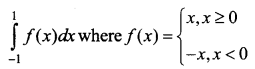Solution: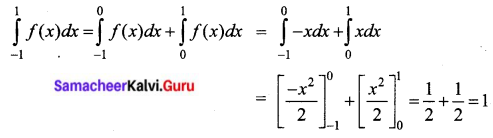Question 4.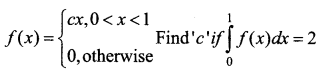Solution: